# [美食话题] 丁香医生科普：扒了50种豆制品，发现豆腐的营养不算啥，最值得吃的是......发表于 2020-11-17 11:37 | 显示全部楼层 |阅读模式

4 P" f0 O! [( J3 r% i(来自：濠.滨.论.坛 bbs.０５１３.org - 南通.濠.滨.网)' ?# p' _6 `# t

6 }5 P# Q0 ?  w) j(来自：濠.滨.论.坛 bbs.０５１３.org - 南通.濠.滨.网)3 b* k0 `  Y" B0 I\$ s
& ~+ G6 c  ^4 Z0 ^(来自：濠.滨.论.坛 bbs.０５１３.org - 南通.濠.滨.网)0 Z) p# v7 W9 x

8 A/ L* x9 ]; b7 f2 x/ ](来自：濠.滨.论.坛 bbs.０５１３.org - 南通.濠.滨.网)- G/ w1 j8 d  b1 I

8 ^% k7 e! H1 {) U- f6 O(来自：濠.滨.论.坛 bbs.０５１３.org - 南通.濠.滨.网)! u\$ D) P: k) C' L  q1 z
" a, L4 H# _: h(来自：濠.滨.论.坛 bbs.０５１３.org - 南通.濠.滨.网)\$ b4 C( }! m" ~/ L# w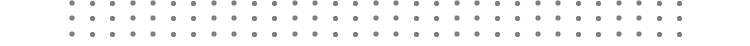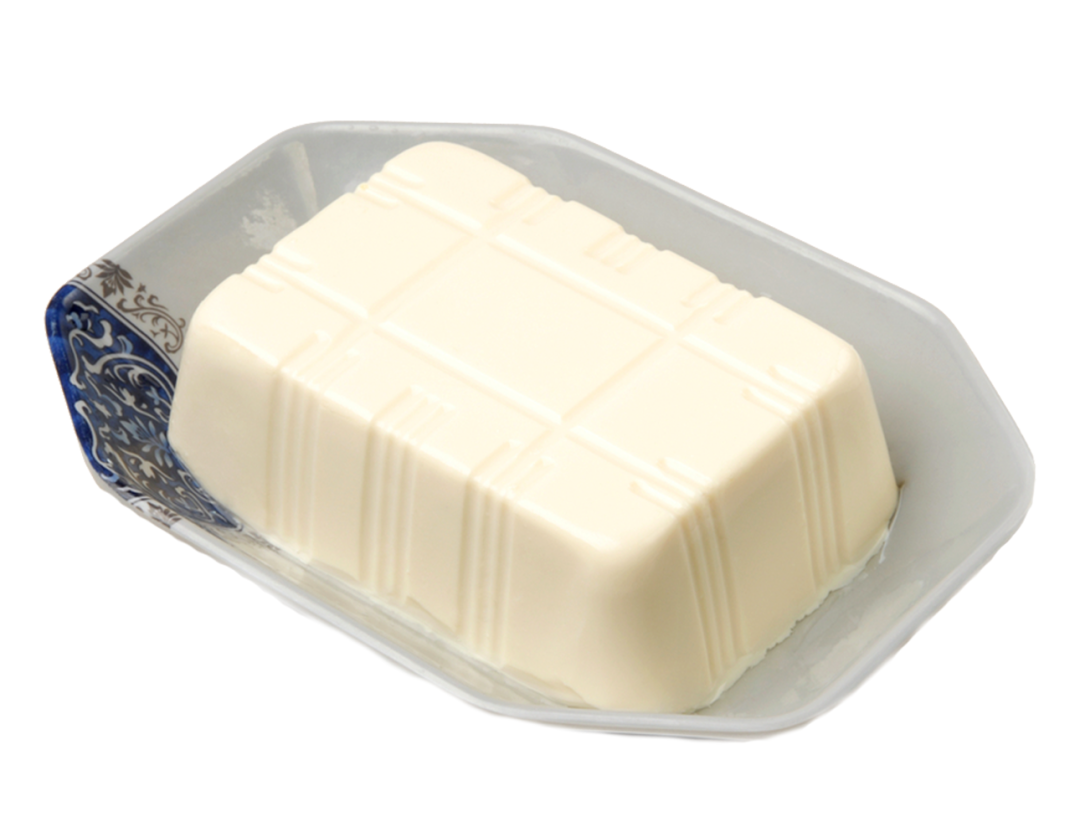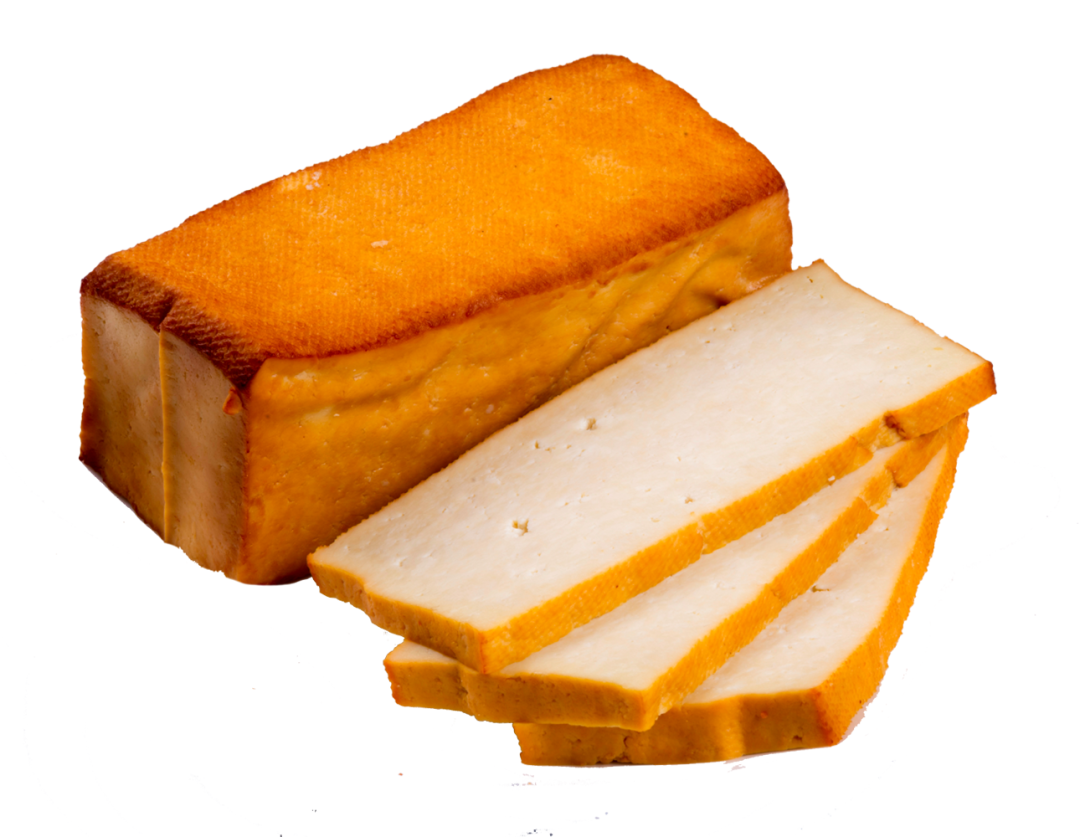每 100 克 内脂豆腐 豆腐干 蛋白质 5.0 克 14.9 克 ???? 钙 17 毫克 447 毫克 ????6 R! h0 h" D# W(来自：濠.滨.论.坛 bbs.０５１３.org - 南通.濠.滨.网)8 [/ \. `( Y9 o* P; C4 h
9 ~! `& f! z  j* K(来自：濠.滨.论.坛 bbs.０５１３.org - 南通.濠.滨.网)+ U; O! ^# k) _  C

' T! r! U3 T* V- K(来自：濠.滨.论.坛 bbs.０５１３.org - 南通.濠.滨.网)4 H: N( B/ l0 ^
\$ L2 H/ f\$ X& q' s(来自：濠.滨.论.坛 bbs.０５１３.org - 南通.濠.滨.网). M! B+ H! {5 Q, x& h

( i, F) l) i# V) |(来自：濠.滨.论.坛 bbs.０５１３.org - 南通.濠.滨.网); g1 N7 ?, K% I; a9 z
5 H& {7 |# D( ]( h(来自：濠.滨.论.坛 bbs.０５１３.org - 南通.濠.滨.网)" X; A; ^5 M& M* J

2 G# Q3 v/ }( A4 [. p(来自：濠.滨.论.坛 bbs.０５１３.org - 南通.濠.滨.网)4 C; t  p: d0 e; s
, E, \  t5 u! d(来自：濠.滨.论.坛 bbs.０５１３.org - 南通.濠.滨.网)! z5 V4 f- A) x  ~" C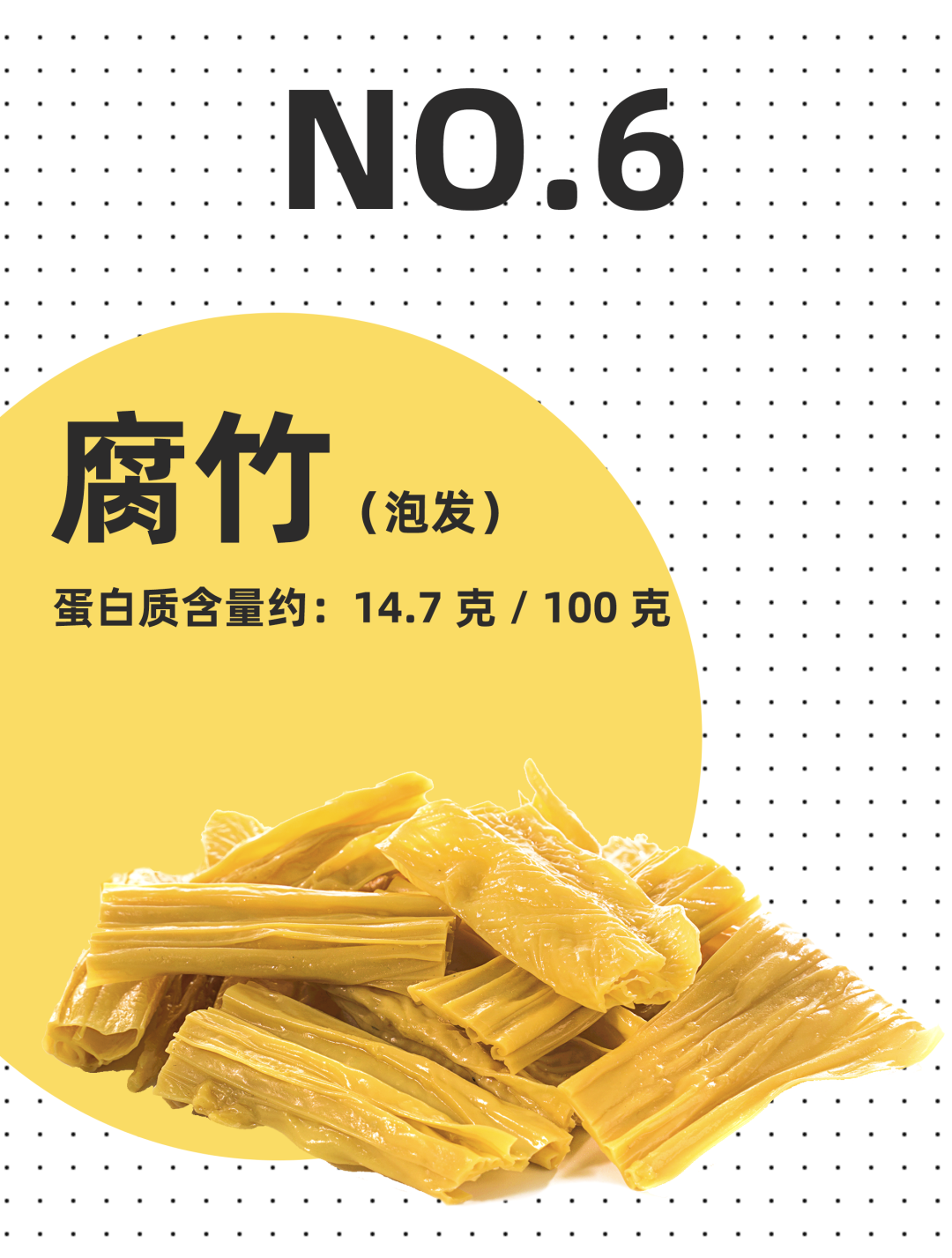4 I. L+ M/ w  p(来自：濠.滨.论.坛 bbs.０５１３.org - 南通.濠.滨.网)6 q4 x; d. n2 M1 v' g
' _5 T1 I0 k" @7 z(来自：濠.滨.论.坛 bbs.０５１３.org - 南通.濠.滨.网)5 ~; w6 E2 U: a3 A

0 m  l( w, Y& f) m(来自：濠.滨.论.坛 bbs.０５１３.org - 南通.濠.滨.网)2 M# q2 O5 y' H3 W

& u- g  j# c4 T(来自：濠.滨.论.坛 bbs.０５１３.org - 南通.濠.滨.网): k9 d- }( v' `

4 S/ y) C5 {- I/ ](来自：濠.滨.论.坛 bbs.０５１３.org - 南通.濠.滨.网)7 \, x# _# I: U5 @
\$ u5 h2 S; w0 P8 m, y(来自：濠.滨.论.坛 bbs.０５１３.org - 南通.濠.滨.网)8 \8 K6 j) f\$ {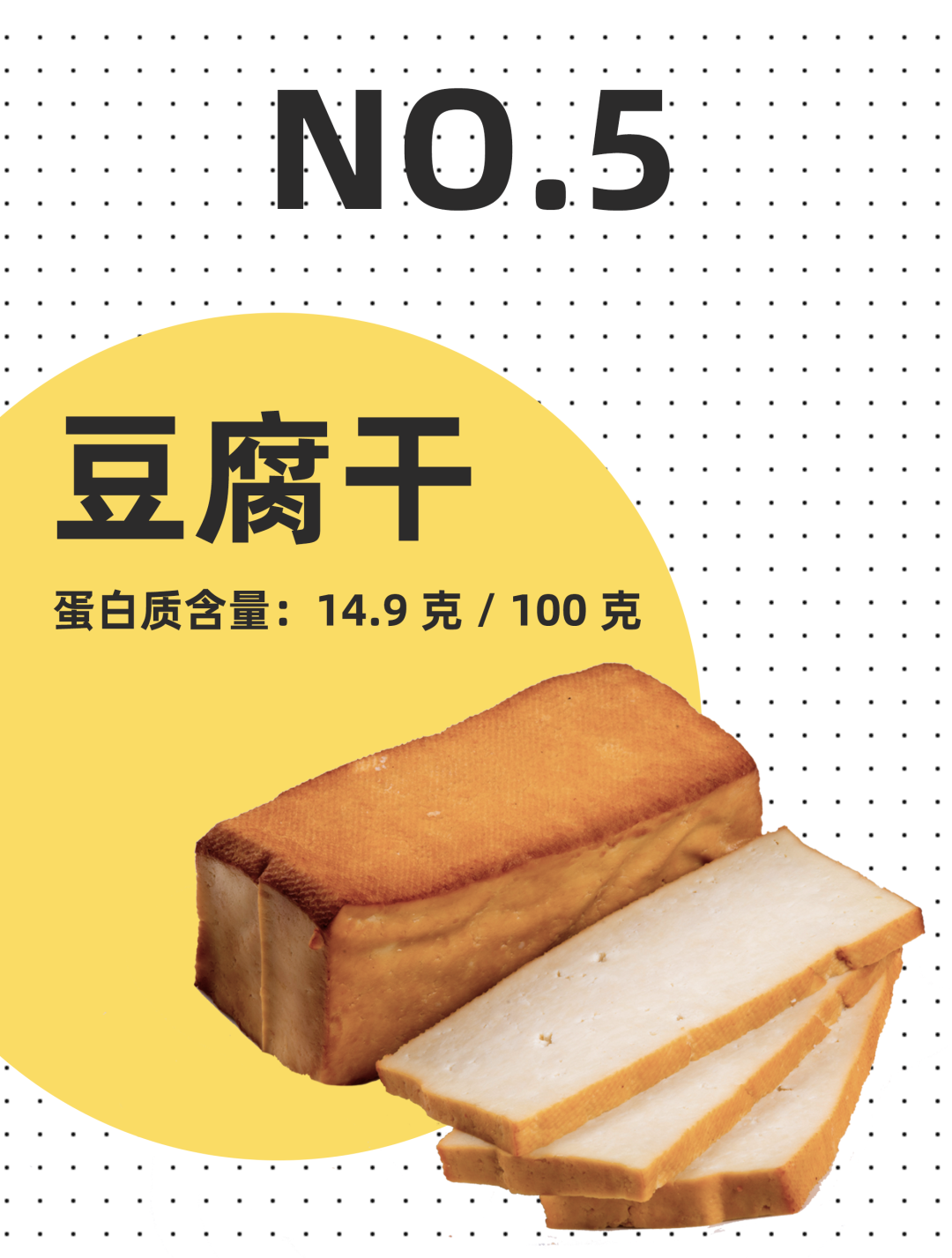" s7 _) O9 e: l(来自：濠.滨.论.坛 bbs.０５１３.org - 南通.濠.滨.网)1 @+ u/ Y' v% i/ v
% l% H6 s  R/ H/ S7 K(来自：濠.滨.论.坛 bbs.０５１３.org - 南通.濠.滨.网)7 a" m2 p! i2 ~/ f0 \

' y8 y/ f; A  }(来自：濠.滨.论.坛 bbs.０５１３.org - 南通.濠.滨.网)- Z7 n+ d4 u5 G! ^

7 d( X' O\$ k, M5 K( Q: Z(来自：濠.滨.论.坛 bbs.０５１３.org - 南通.濠.滨.网)3 E7 `9 J; s9 i

& d- t2 m: z& ?0 U( v(来自：濠.滨.论.坛 bbs.０５１３.org - 南通.濠.滨.网): e& Q; U! ^, p- @

+ {: w. U+ ^# ?(来自：濠.滨.论.坛 bbs.０５１３.org - 南通.濠.滨.网)+ x( n\$ X* N, v3 a+ @
0 X- u, h8 z+ t3 S6 \(来自：濠.滨.论.坛 bbs.０５１３.org - 南通.濠.滨.网)\$ O* ]( _5 Q1 g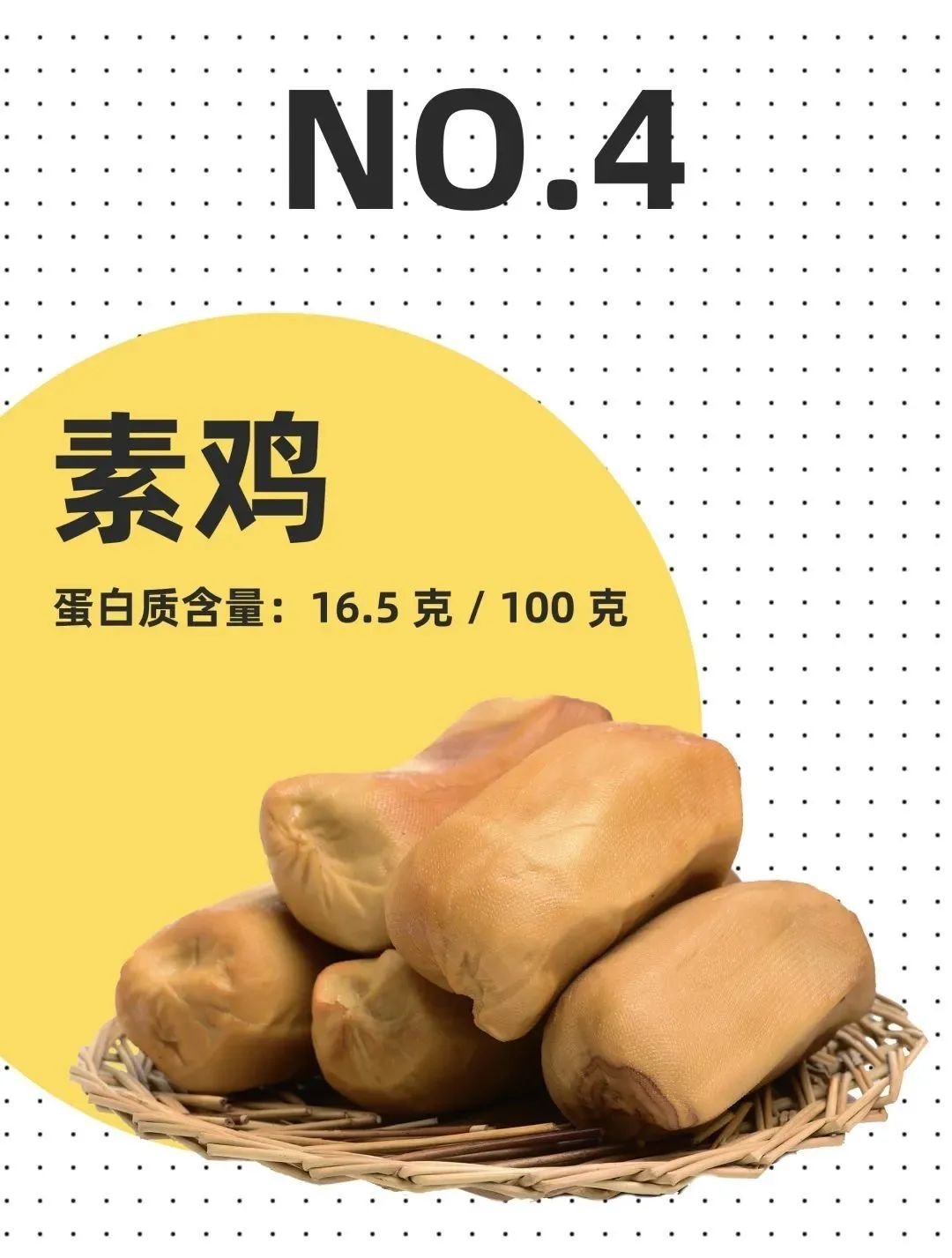\$ K5 q6 D8 p( I3 }(来自：濠.滨.论.坛 bbs.０５１３.org - 南通.濠.滨.网)3 K% V% @; O1 H
. ]  ?8 |# x\$ o(来自：濠.滨.论.坛 bbs.０５１３.org - 南通.濠.滨.网)5 S0 e; G1 U# ^6 p! k

6 R3 E2 t+ L) ~5 p(来自：濠.滨.论.坛 bbs.０５１３.org - 南通.濠.滨.网); _( Z! J# o8 F

3 I( L/ j+ F* @" L(来自：濠.滨.论.坛 bbs.０５１３.org - 南通.濠.滨.网)3 }2 [: k  r* \' D
0 z; ~3 C% P, t, H0 U(来自：濠.滨.论.坛 bbs.０５１３.org - 南通.濠.滨.网)3 B5 z8 D) t- k: h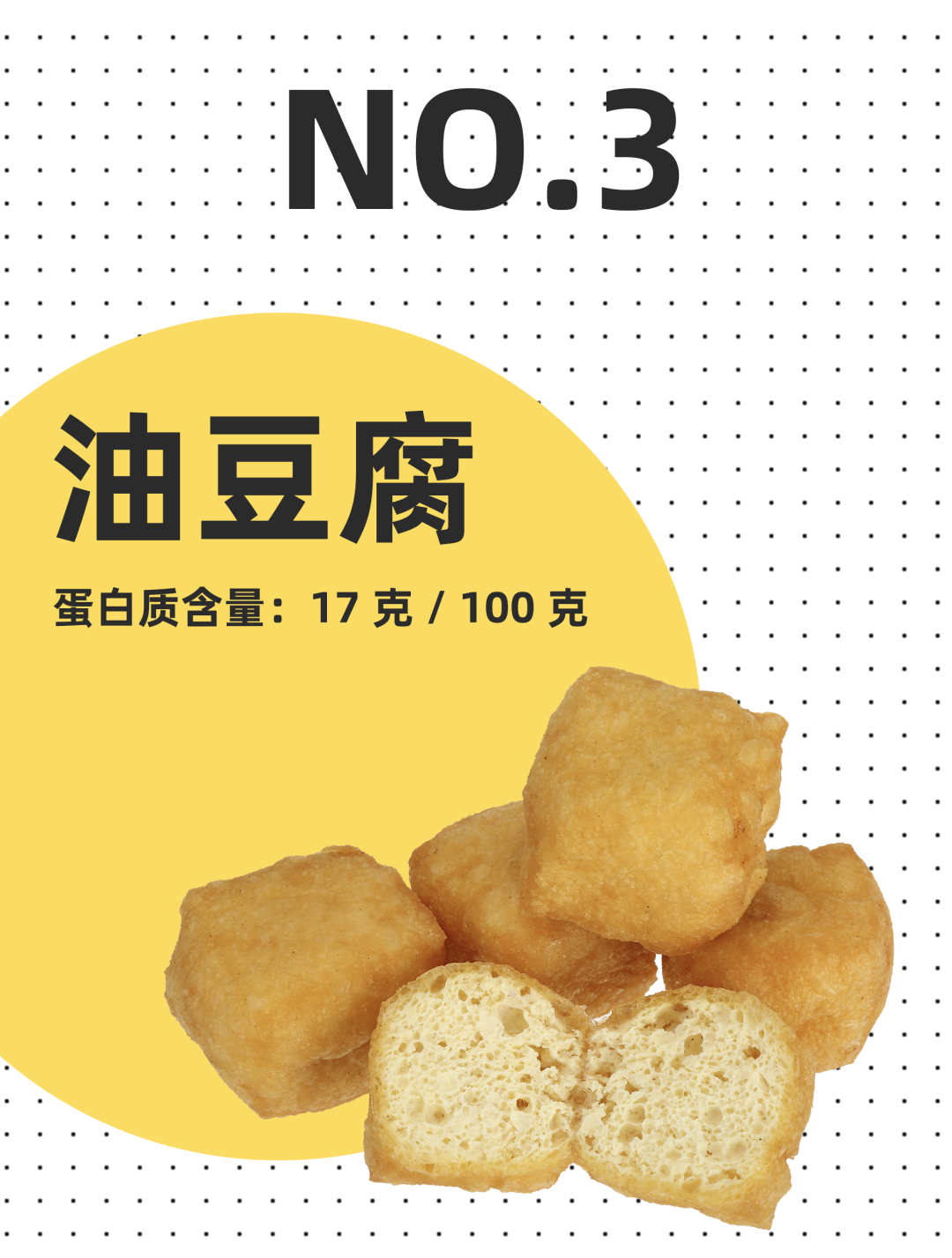: h! {( s/ B' t; c(来自：濠.滨.论.坛 bbs.０５１３.org - 南通.濠.滨.网)) N5 J9 v2 l, A! F! q

) q& q9 M# o1 v0 [(来自：濠.滨.论.坛 bbs.０５１３.org - 南通.濠.滨.网)8 B# \\$ h; q+ U" }

' h+ W; h; R\$ X: T(来自：濠.滨.论.坛 bbs.０５１３.org - 南通.濠.滨.网)9 T0 T1 X5 `- Y' I\$ r
3 Y5 o+ ^1 n1 S(来自：濠.滨.论.坛 bbs.０５１３.org - 南通.濠.滨.网)4 b1 C  X7 g\$ g, t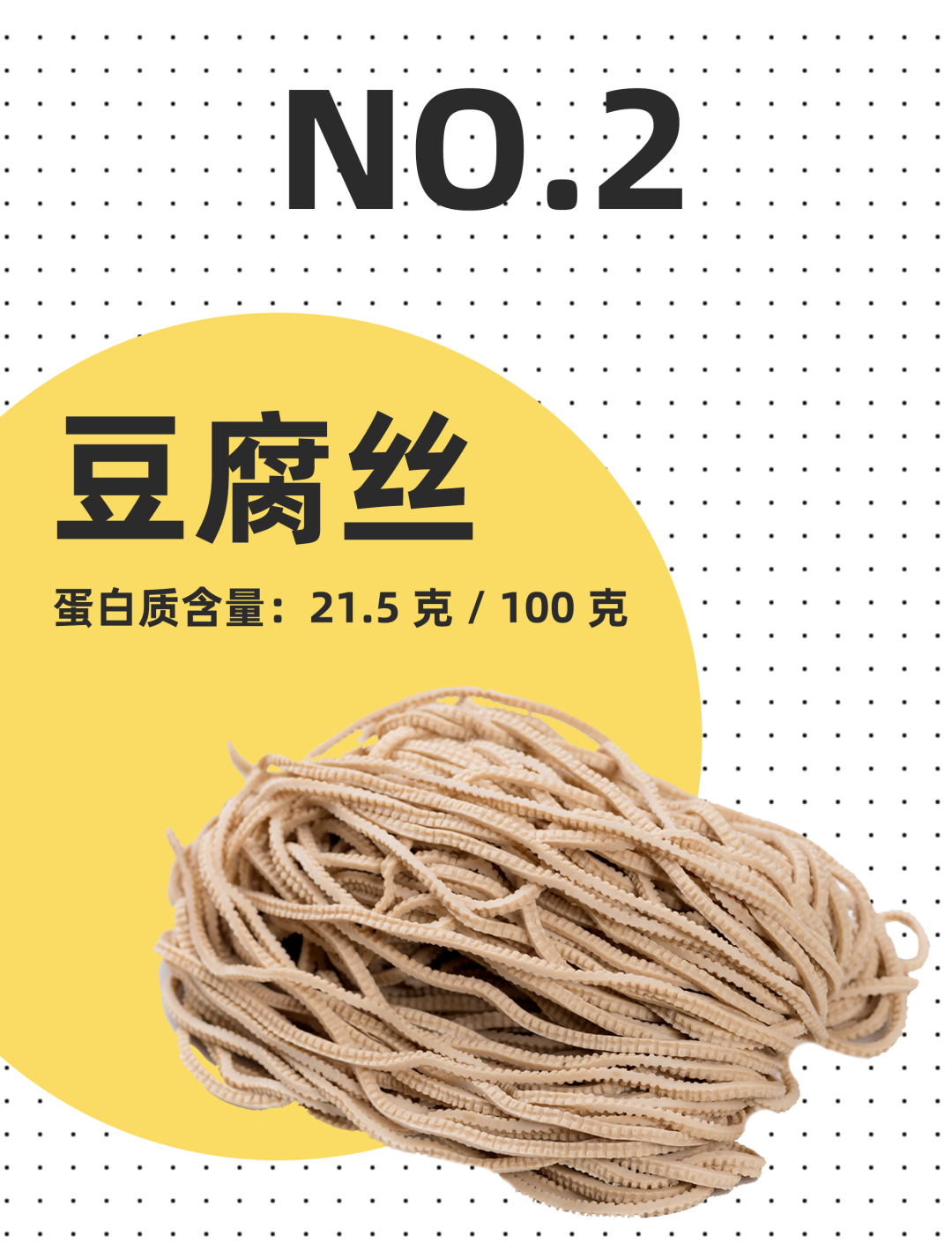: z7 I7 `' R+ V(来自：濠.滨.论.坛 bbs.０５１３.org - 南通.濠.滨.网)\$ D" r  k+ E* Q
X! @. l1 e9 f* W0 s) X(来自：濠.滨.论.坛 bbs.０５１３.org - 南通.濠.滨.网)" p9 D* G% R9 [, Y

3 A- k# @\$ E' V' a(来自：濠.滨.论.坛 bbs.０５１３.org - 南通.濠.滨.网): O( o9 r% Y2 z% y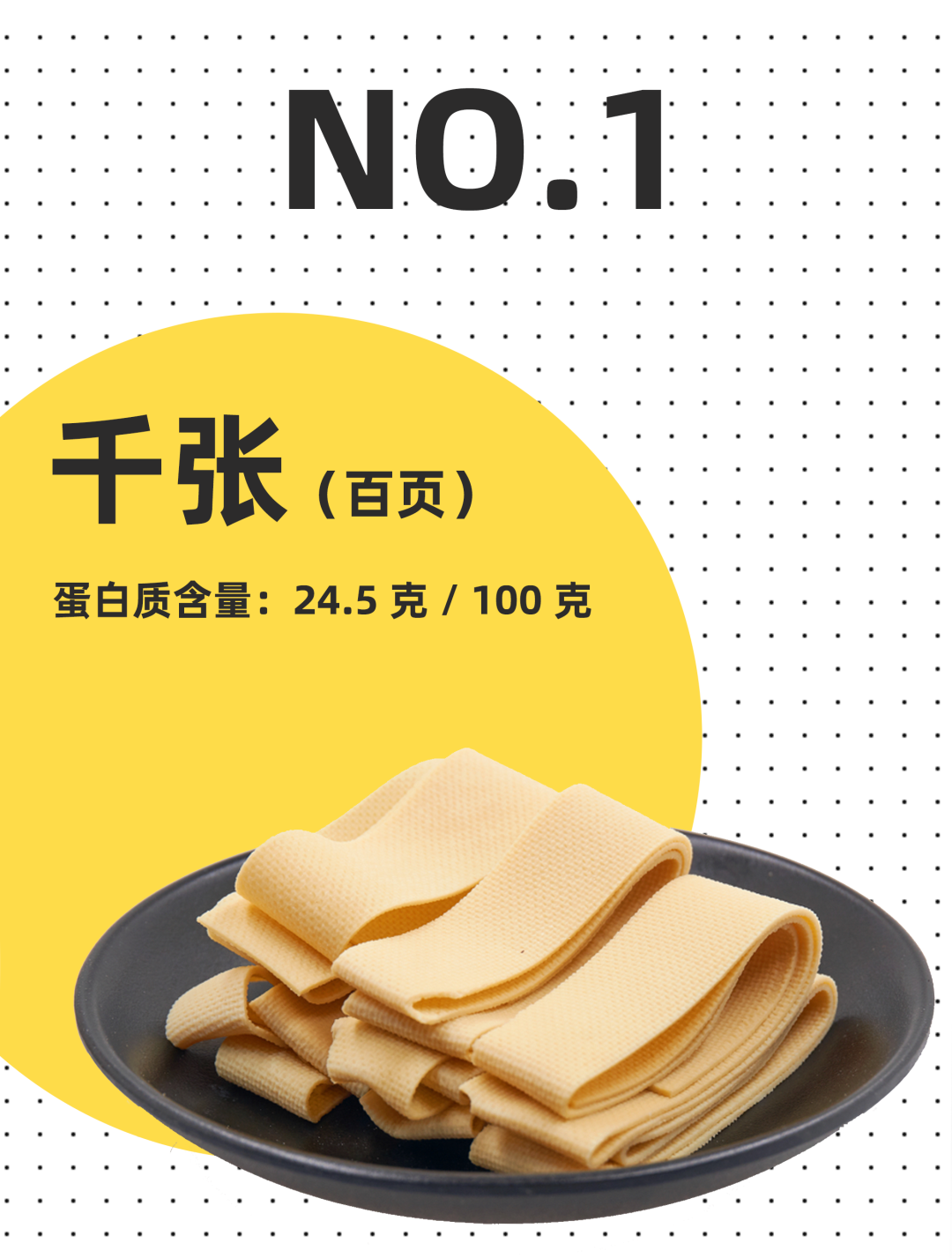k* @8 J: P, w9 I(来自：濠.滨.论.坛 bbs.０５１３.org - 南通.濠.滨.网)1 r2 U/ Q6 s! `% F

# m2 l0 X' o6 N(来自：濠.滨.论.坛 bbs.０５１３.org - 南通.濠.滨.网)) ~; K1 m1 x) x4 v

* L' v( d. m' ^(来自：濠.滨.论.坛 bbs.０５１３.org - 南通.濠.滨.网)8 D- f" f* T7 d. p

6 X) `8 ?5 @% D4 m7 P(来自：濠.滨.论.坛 bbs.０５１３.org - 南通.濠.滨.网)* p- S: \! g% G. N

4 L  ~. m4 G* r% ?+ R: U(来自：濠.滨.论.坛 bbs.０５１３.org - 南通.濠.滨.网): G% B0 z, i8 I
/ ^: Q1 v/ \! t& t(来自：濠.滨.论.坛 bbs.０５１３.org - 南通.濠.滨.网)% ^5 h/ e& |2 U\$ b
0 A- R) @( d\$ u! P(来自：濠.滨.论.坛 bbs.０５１３.org - 南通.濠.滨.网)* X( x+ G3 }' R\$ D% O# j

( _, t4 E+ G: A1 ?(来自：濠.滨.论.坛 bbs.０５１３.org - 南通.濠.滨.网)) p& `; v( [, B8 U
+ @# F9 W. a& L(来自：濠.滨.论.坛 bbs.０５１３.org - 南通.濠.滨.网)( i! r% |, r* N4 \

( c5 w! K) B\$ b: J(来自：濠.滨.论.坛 bbs.０５１３.org - 南通.濠.滨.网)\$ L; c1 t. Q( [  X" x

8 W5 y5 P/ O) q1 P1 |(来自：濠.滨.论.坛 bbs.０５１３.org - 南通.濠.滨.网)/ |" ]" a& w9 P. e

; x- K3 O: V% m; R: c. n(来自：濠.滨.论.坛 bbs.０５１３.org - 南通.濠.滨.网)1 j0 P8 O5 Z# g0 o
( U\$ e. F) K* F) H(来自：濠.滨.论.坛 bbs.０５１３.org - 南通.濠.滨.网)8 c! i/ ?2 S! O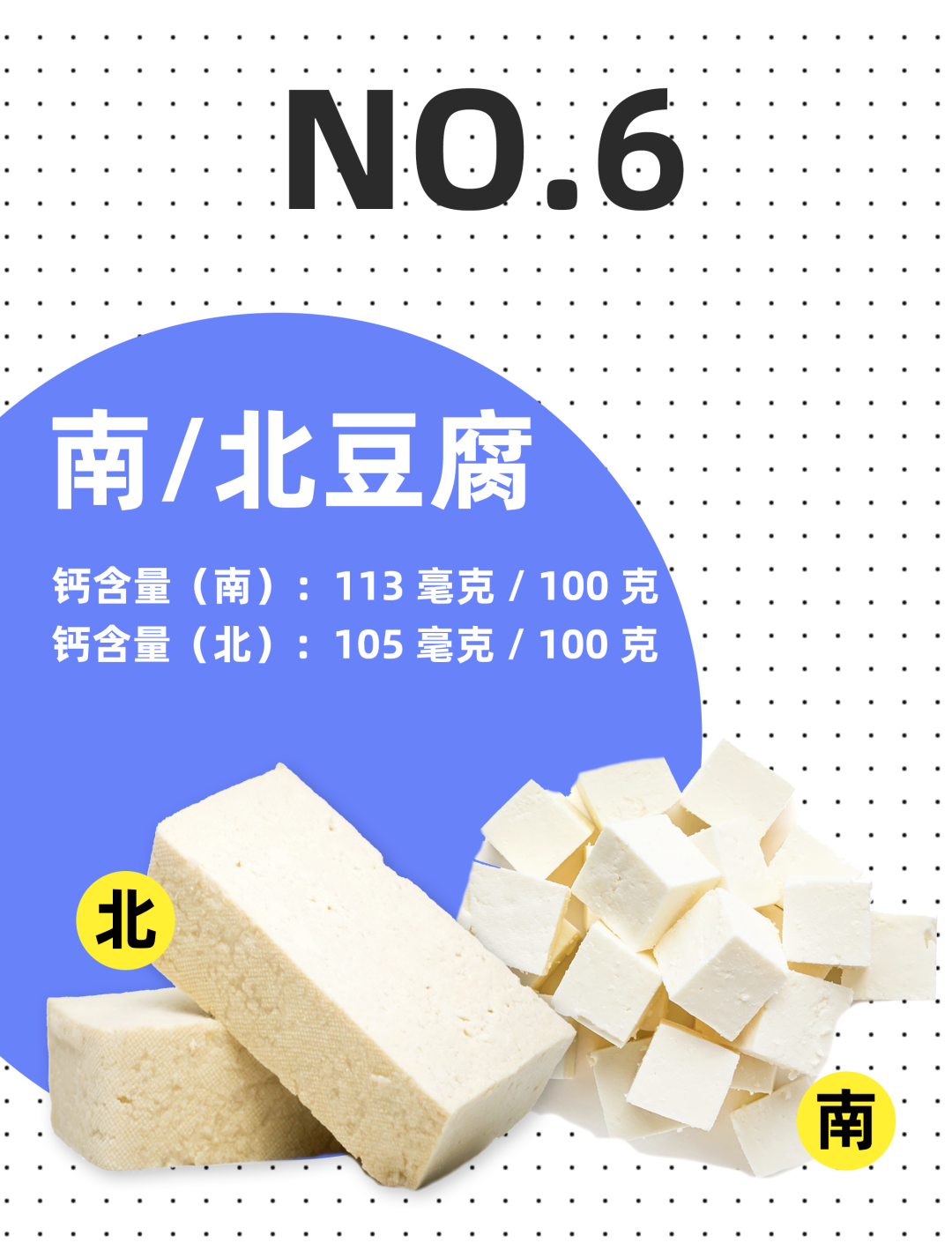( H' H6 x4 B! u2 S(来自：濠.滨.论.坛 bbs.０５１３.org - 南通.濠.滨.网)+ N3 J# Q2 q. ~* i
! p* r! M1 v0 \* o(来自：濠.滨.论.坛 bbs.０５１３.org - 南通.濠.滨.网)4 j\$ L. Y  {6 ~

x( w0 w8 h) }) p' w(来自：濠.滨.论.坛 bbs.０５１３.org - 南通.濠.滨.网), K0 A2 ]9 j3 f7 U

: z) Z6 }7 l9 `(来自：濠.滨.论.坛 bbs.０５１３.org - 南通.濠.滨.网)2 }2 }% h# D' ?4 ?
0 ^5 I. j; B3 a1 Y& Q(来自：濠.滨.论.坛 bbs.０５１３.org - 南通.濠.滨.网)- k2 ?/ M) z3 C& e" n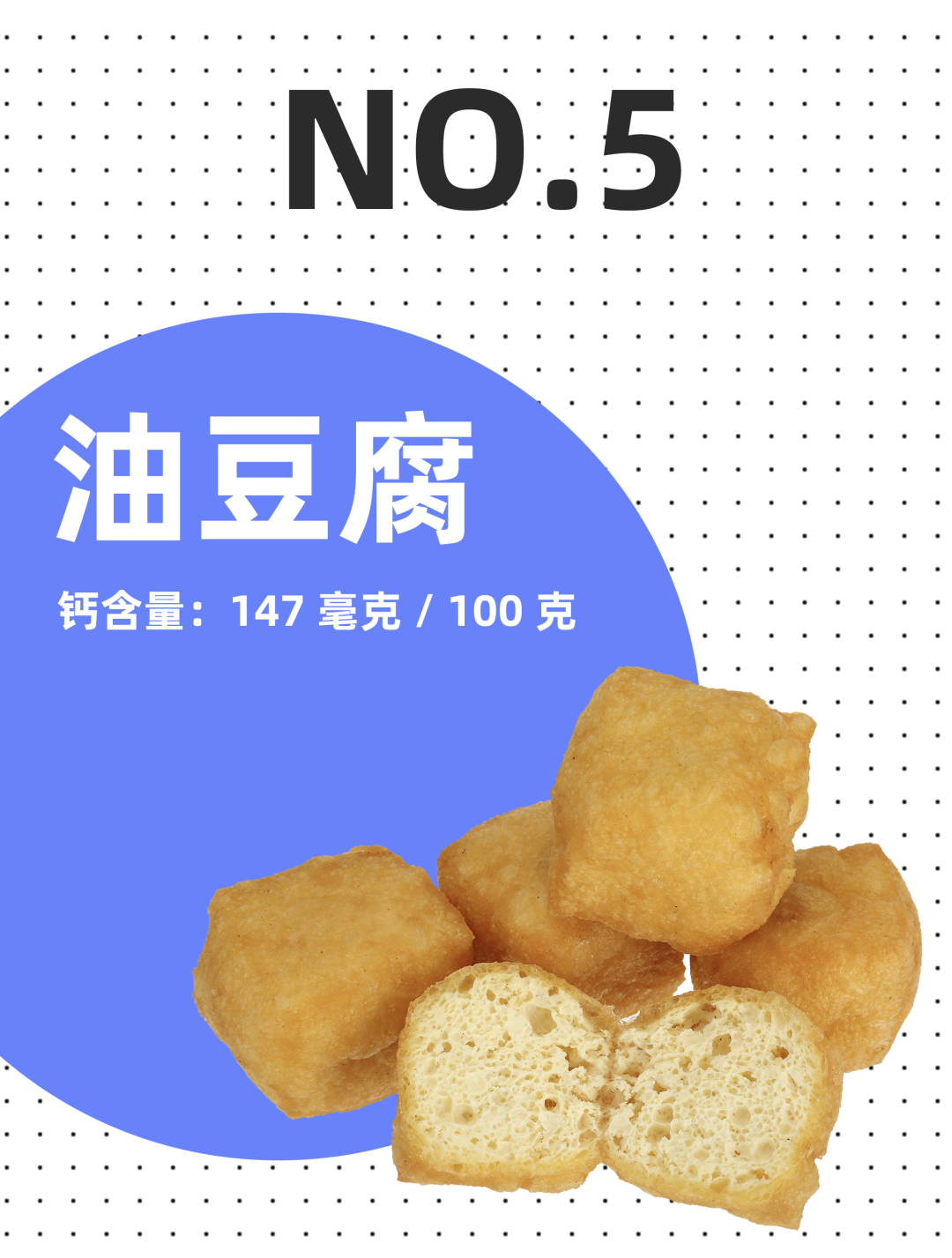& s3 E- |9 `* t9 k(来自：濠.滨.论.坛 bbs.０５１３.org - 南通.濠.滨.网)# B' v3 q; m; c' L# B
+ C" w. L, v) e' B4 Q(来自：濠.滨.论.坛 bbs.０５１３.org - 南通.濠.滨.网)  A8 h4 r' b2 M5 o' H

1 A5 `1 m, P2 Y6 _0 T(来自：濠.滨.论.坛 bbs.０５１３.org - 南通.濠.滨.网)2 a0 a* |9 ^" U% K. v

! z# @\$ T. i1 n* I1 `(来自：濠.滨.论.坛 bbs.０５１３.org - 南通.濠.滨.网); b, K  p4 U5 e1 r

- M* j\$ [7 G6 m. Z(来自：濠.滨.论.坛 bbs.０５１３.org - 南通.濠.滨.网)7 n: R1 ~  r0 @
; {% A) S9 r: Q" i0 T0 J(来自：濠.滨.论.坛 bbs.０５１３.org - 南通.濠.滨.网)# O  M* j5 X  n' F. b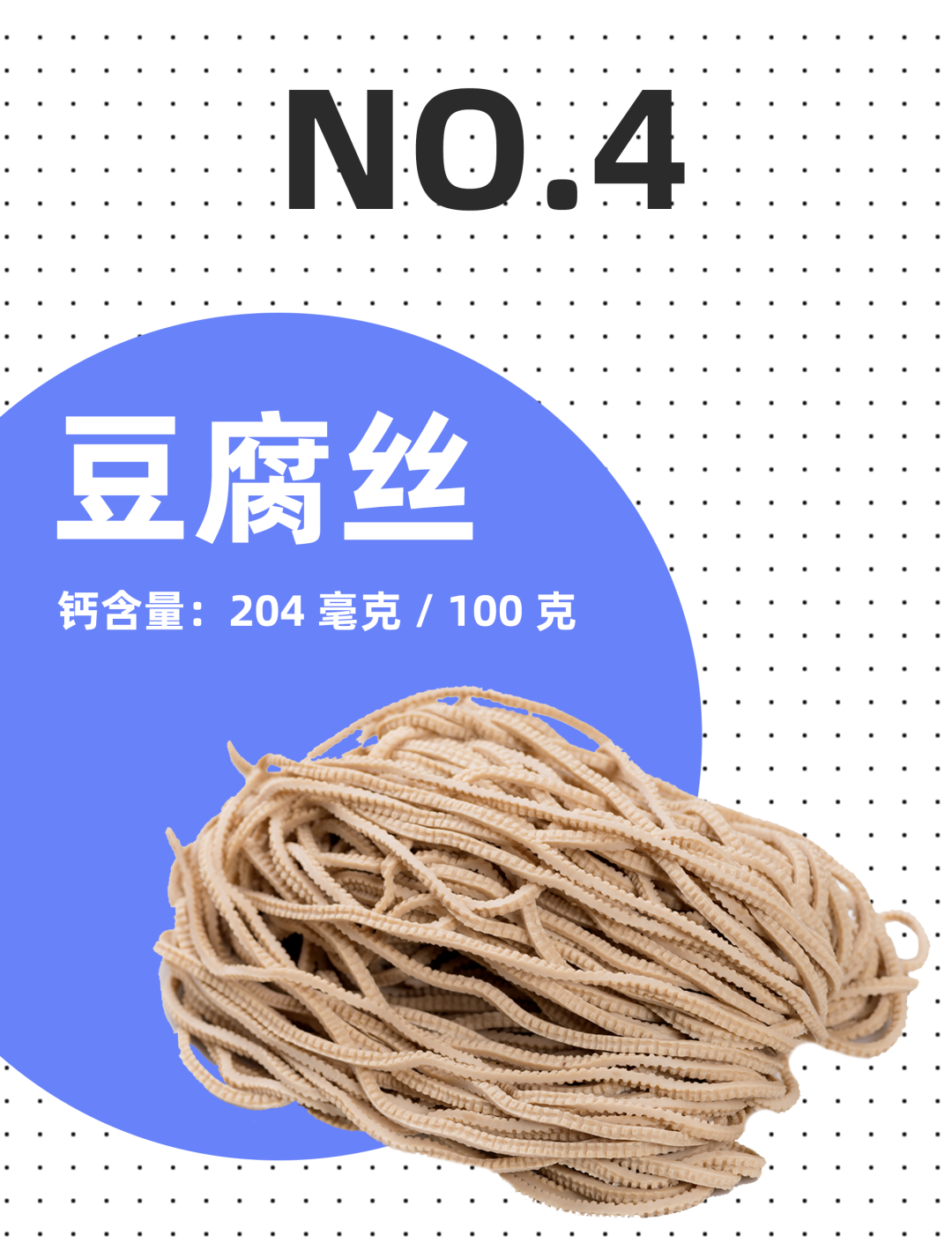# S! h9 x& X1 G; u' Z(来自：濠.滨.论.坛 bbs.０５１３.org - 南通.濠.滨.网): Z; V4 {9 Y# v) Q
' @( P  s+ G\$ Q(来自：濠.滨.论.坛 bbs.０５１３.org - 南通.濠.滨.网)  c# d; B/ z/ j/ _- T

+ T, e  P* B8 h' e(来自：濠.滨.论.坛 bbs.０５１３.org - 南通.濠.滨.网)8 [/ t, t4 U+ `) p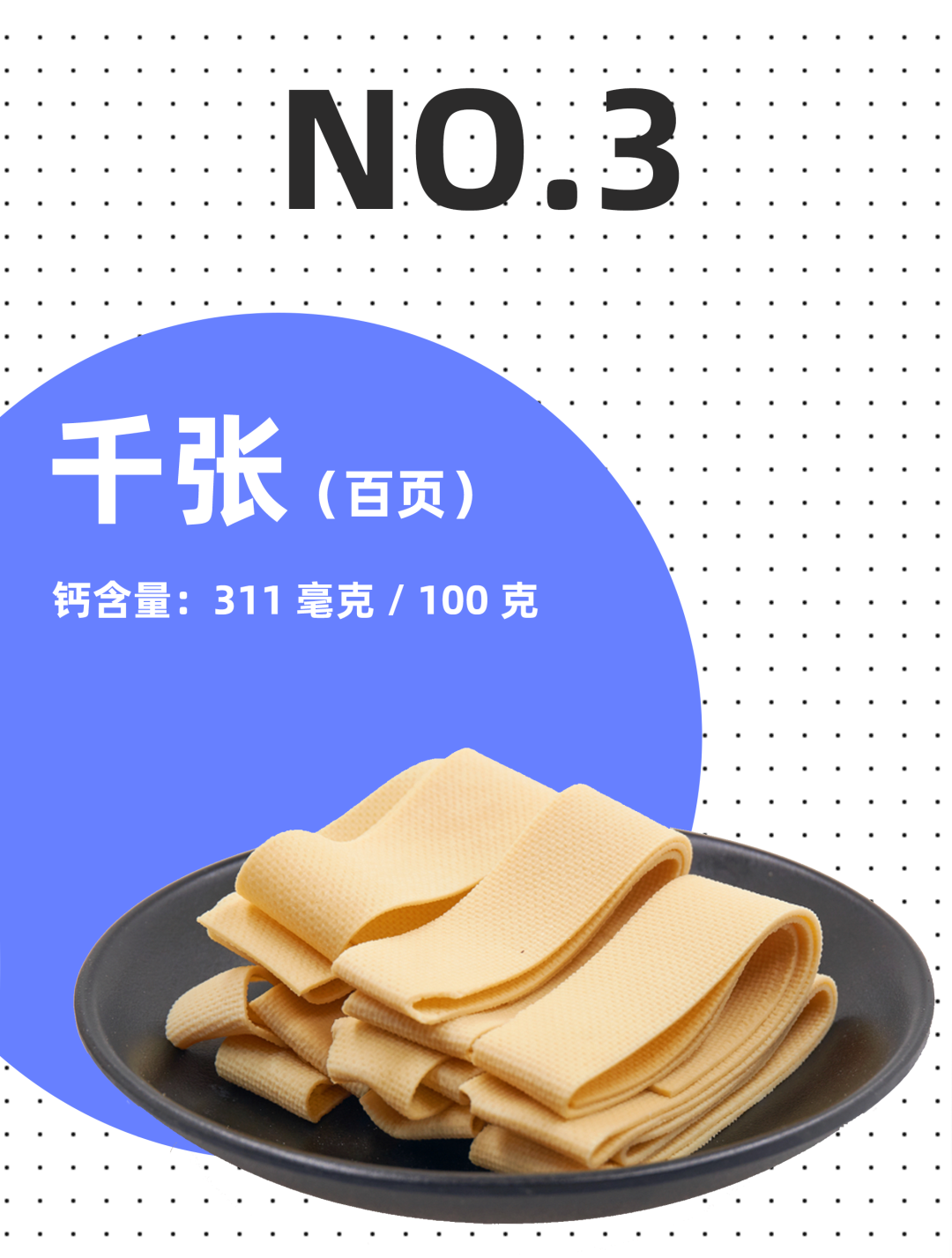; L9 L5 R6 _\$ N( _5 Q(来自：濠.滨.论.坛 bbs.０５１３.org - 南通.濠.滨.网)% _: U1 O! ]( u
; s1 w! z- u' U% [(来自：濠.滨.论.坛 bbs.０５１３.org - 南通.濠.滨.网)6 _# R* _, t1 i/ r: v( H

) W2 u( v\$ M1 u(来自：濠.滨.论.坛 bbs.０５１３.org - 南通.濠.滨.网)8 O- J) s, c6 f& p5 v

* ^0 ?1 |9 }\$ A! j: n7 [(来自：濠.滨.论.坛 bbs.０５１３.org - 南通.濠.滨.网)" {* t\$ l3 P5 Y( \* k

' M: @+ w0 G6 ^0 X1 y(来自：濠.滨.论.坛 bbs.０５１３.org - 南通.濠.滨.网). Z1 d& d: }: J
" ~8 R5 u3 `\$ \\$ a(来自：濠.滨.论.坛 bbs.０５１３.org - 南通.濠.滨.网)5 r" F7 h# n; e% u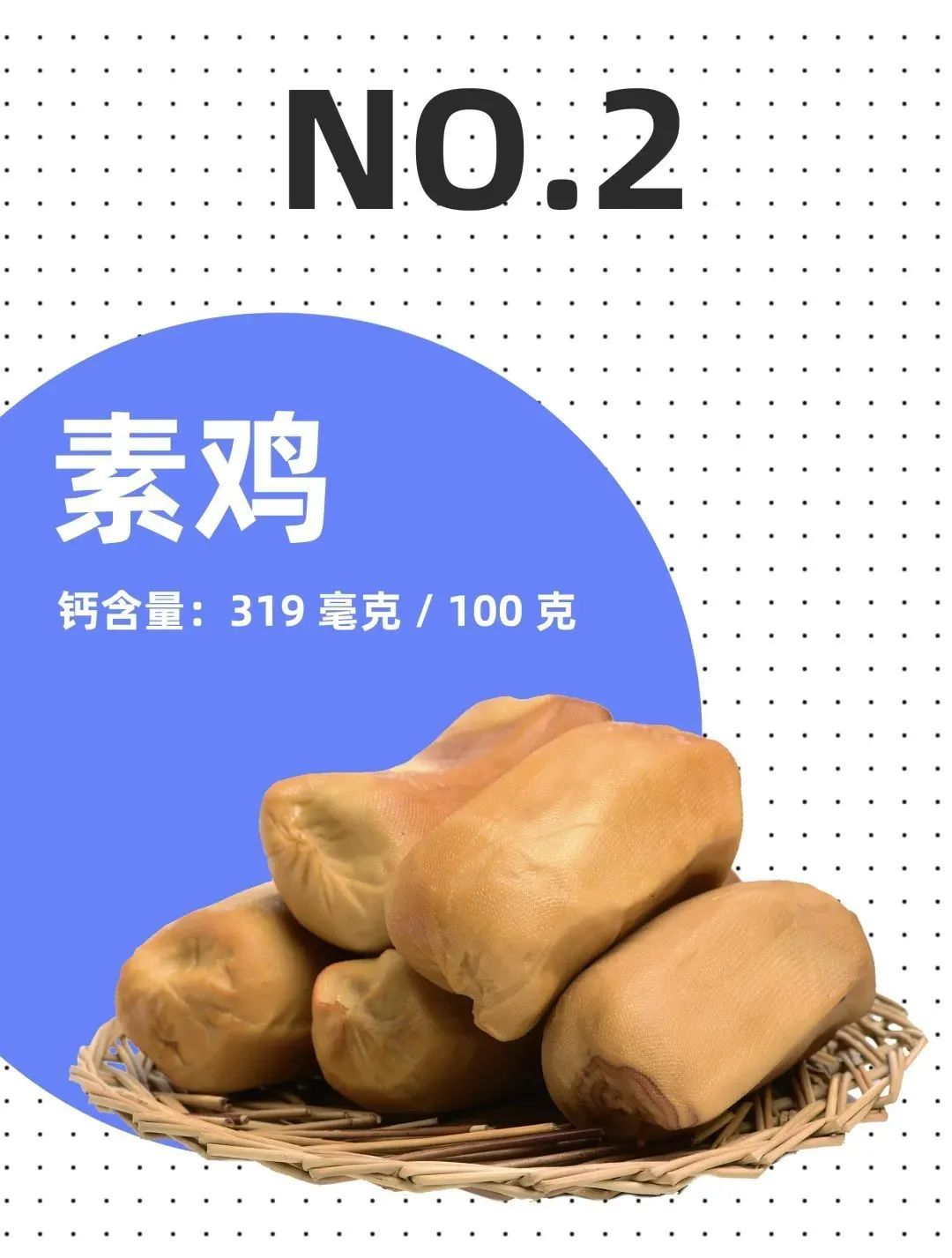9 h/ q) Z\$ ]8 |) q+ W% t(来自：濠.滨.论.坛 bbs.０５１３.org - 南通.濠.滨.网)5 T7 l1 X# G" ^  Q
& w( M4 C9 r7 O(来自：濠.滨.论.坛 bbs.０５１３.org - 南通.濠.滨.网)3 }5 r5 F: F# i5 N2 F& W

3 k" I* g) h) l  u(来自：濠.滨.论.坛 bbs.０５１３.org - 南通.濠.滨.网)4 F( d+ ^( A" Z\$ k* p0 V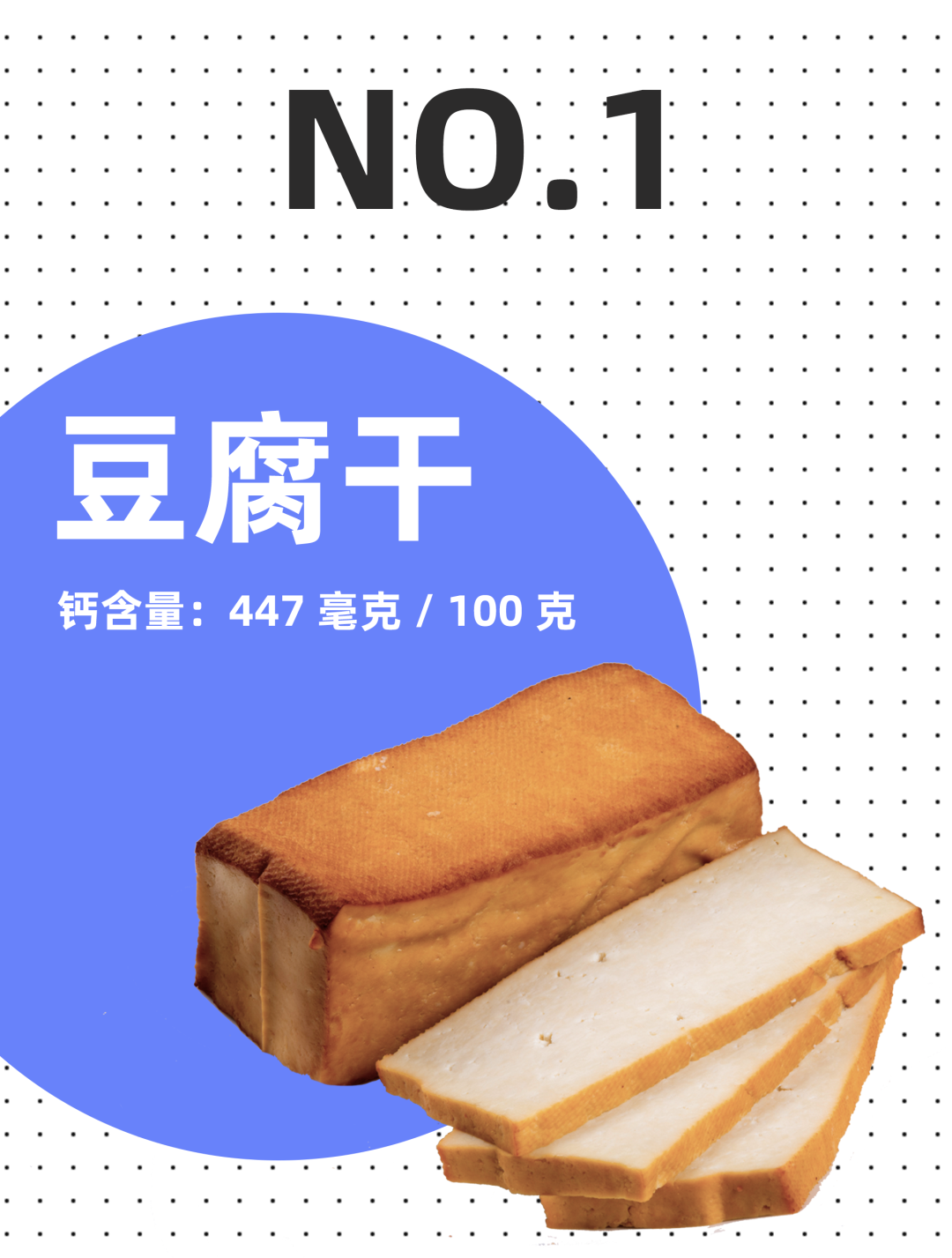/ k; ~7 H& f' k\$ i(来自：濠.滨.论.坛 bbs.０５１３.org - 南通.濠.滨.网)3 q/ H1 j4 H* Z# s' m& N
5 v1 l/ T5 r3 I) B! ~(来自：濠.滨.论.坛 bbs.０５１３.org - 南通.濠.滨.网)  X0 K4 _6 t/ L7 k\$ z

/ Z+ j: d' F: m, s(来自：濠.滨.论.坛 bbs.０５１３.org - 南通.濠.滨.网)' }\$ ?# w9 j) Q

6 R% b) h- P. k; H(来自：濠.滨.论.坛 bbs.０５１３.org - 南通.濠.滨.网)) F7 B  s9 |  W( n+ s+ P

" g1 Z7 j% q2 g0 J/ g5 r(来自：濠.滨.论.坛 bbs.０５１３.org - 南通.濠.滨.网)5 U; J\$ L: \3 R; Z
????

6 A' H+ x6 V- L9 \(来自：濠.滨.论.坛 bbs.０５１３.org - 南通.濠.滨.网)4 {) O# [! d% a
- i+ Y; Y+ I\$ u: x6 G(来自：濠.滨.论.坛 bbs.０５１３.org - 南通.濠.滨.网): {) C5 [6 j  C1 c" f) p

# `  M% b& N: p9 W5 a(来自：濠.滨.论.坛 bbs.０５１３.org - 南通.濠.滨.网)1 }1 Y* t' F! \9 B

. @, D\$ ^, t# k(来自：濠.滨.论.坛 bbs.０５１３.org - 南通.濠.滨.网); R0 N7 \1 H2 B
 中国营养学会 中国食物成分表
 葛淑华,刁屾,栾俊,王雪,袁燕,王全杰.蛋白质螯合金属研究进展及应用[J].皮革与化工,2018,35(02):21-25.
 林洪斌. 豆腐干质量控制关键工艺研究[D].西华大学,2013.
 张红梅,顾和平,陈华涛,袁星星,崔晓艳,陈新.国内外豆腐加工主要研究进展[J].江苏农业科学,2010(05):409-410.
 李永飞,彭昌亚,孙英鹏,吕季璋.传统豆腐干生产工艺的改进措施[J].农产品加工,2007(01):42-43.
 汪永辉.芜湖千张的制作[J].农家科技,1996(11):37.
 苏更林.素鸡的制作[J].河北农业科技,1996(02):34-35.

" r) [. V& Q+ a3 r(来自：濠.滨.论.坛 bbs.０５１３.org - 南通.濠.滨.网)% {7 i+ H1 ^1 D/ j3 ]发表于 2020-11-17 16:19 | 显示全部楼层
 脂肪含量呢？哪个最低

 您需要登录后才可以回帖 登录 | 注册 本版积分规则 回帖并转播 回帖后跳转到最后一页